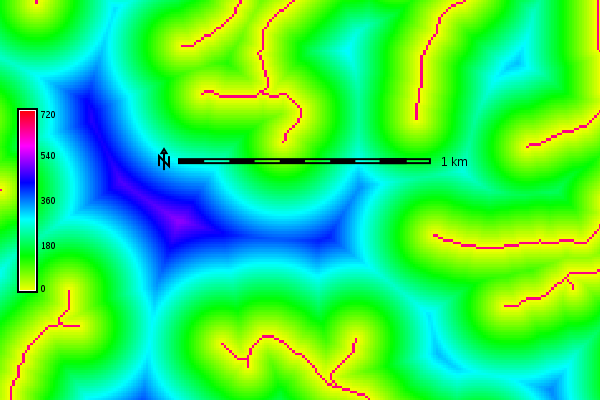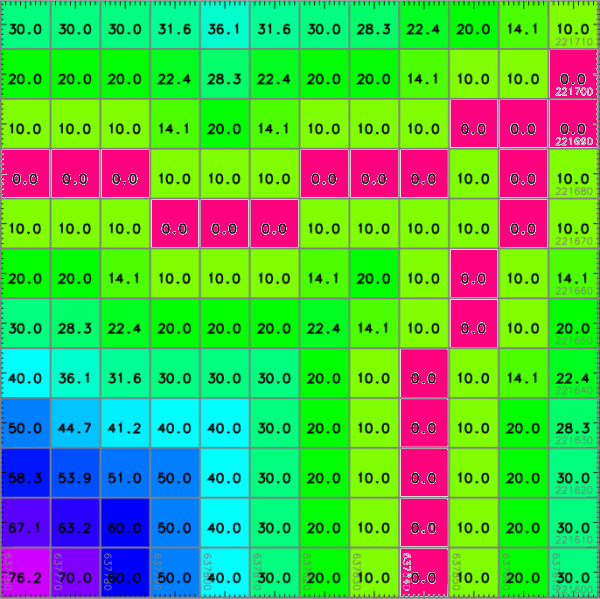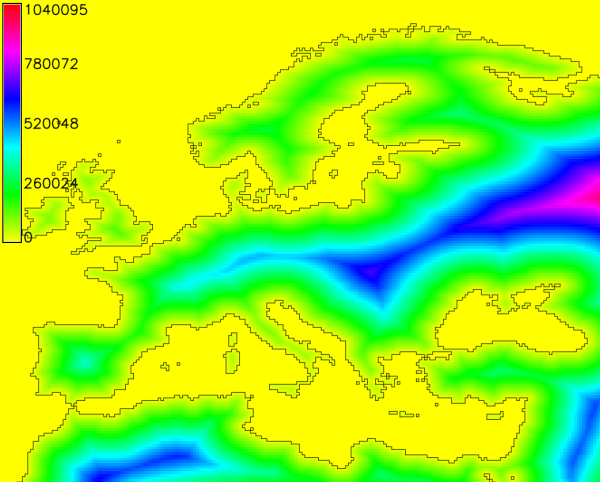Note: A new GRASS GIS stable version has been released: GRASS GIS 7.8, available here.
Updated manual page: here

## NAME

r.grow.distance - Generates a raster map containing distances to nearest raster features.

## KEYWORDS

raster, distance, proximity

## SYNOPSIS

r.grow.distance
r.grow.distance --help
r.grow.distance [-mn] input=name [distance=name] [value=name] [metric=string] [--overwrite] [--help] [--verbose] [--quiet] [--ui]

### Flags:

-m
Output distances in meters instead of map units
-n
Calculate distance to nearest NULL cell
--overwrite
Allow output files to overwrite existing files
--help
Print usage summary
--verbose
Verbose module output
--quiet
Quiet module output
--ui
Force launching GUI dialog

### Parameters:

input=name [required]
Name of input raster map
distance=name
Name for distance output raster map
value=name
Name for value output raster map
metric=string
Metric
Options: euclidean, squared, maximum, manhattan, geodesic
Default: euclidean

## DESCRIPTION

r.grow.distance generates raster maps representing the distance to the nearest non-null cell in the input map and/or the value of the nearest non-null cell.

## NOTES

The flag -n calculates the respective pixel distances to the nearest NULL cell.

The user has the option of specifying five different metrics which control the geometry in which grown cells are created, (controlled by the metric parameter): Euclidean, Squared, Manhattan, Maximum, and Geodesic.

The Euclidean distance or Euclidean metric is the "ordinary" distance between two points that one would measure with a ruler, which can be proven by repeated application of the Pythagorean theorem. The formula is given by:

```d(dx,dy) = sqrt(dx^2 + dy^2)
```
Cells grown using this metric would form isolines of distance that are circular from a given point, with the distance given by the radius.

The Squared metric is the Euclidean distance squared, i.e. it simply omits the square-root calculation. This may be faster, and is sufficient if only relative values are required.

The Manhattan metric, or Taxicab geometry, is a form of geometry in which the usual metric of Euclidean geometry is replaced by a new metric in which the distance between two points is the sum of the (absolute) differences of their coordinates. The name alludes to the grid layout of most streets on the island of Manhattan, which causes the shortest path a car could take between two points in the city to have length equal to the points' distance in taxicab geometry. The formula is given by:

```d(dx,dy) = abs(dx) + abs(dy)
```
where cells grown using this metric would form isolines of distance that are rhombus-shaped from a given point.

The Maximum metric is given by the formula

```d(dx,dy) = max(abs(dx),abs(dy))
```
where the isolines of distance from a point are squares.

The Geodesic metric is calculated as geodesic distance, to be used only in latitude-longitude locations. It is recommended to use it along with the -m flag in order to output distances in meters instead of map units.

## EXAMPLES

### Distance from the streams network

North Carolina sample dataset:
```g.region raster=streams_derived -p
r.grow.distance input=streams_derived distance=dist_from_streams
```Euclidean distance from the streams network in meters (map subset)Euclidean distance from the streams network in meters (detail, numbers shown with d.rast.num)

### Distance from sea in meters in latitude-longitude location

```g.region raster=sea -p
r.grow.distance -m input=sea distance=dist_from_sea_geodetic metric=geodesic
```Geodesic distances to sea in meters

r.grow, r.distance, r.buffer, r.cost, r.patch

## AUTHORS

Glynn Clements

Last changed: \$Date\$

## SOURCE CODE

Available at: r.grow.distance source code (history)

Note: A new GRASS GIS stable version has been released: GRASS GIS 7.8, available here.
Updated manual page: here

© 2003-2020 GRASS Development Team, GRASS GIS 7.6.2dev Reference Manual